# Neon

Jump to: navigation, search

Neon (Ne)

## Models

#### Buckingham potential

The Buckingham potential for neon is given by (Eq. 26 ):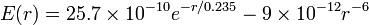$E(r) = 25.7 \times 10^{-10} e^{-r/0.235} -9 \times 10^{-12} r^{-6}$

where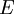$E$ is in ergs ( 10−7 J) and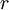$r$ in Å.

#### HBV potential

The Hellmann-Bich-Vogel potential .

#### Lennard-Jones parameters

Some Lennard-Jones parameters for neon are listed in the following table:

 Authors$\epsilon$ (meV)$\sigma$ (nm) Reference Herrero 3.0840 0.2782  Ramírez and Herrero 3.2135 0.2782 

.

.

# Phase diagram

The phase diagram for temperatures in the range of 17–50 K and pressures between 10−2 and 2×103 bar has been calculated in Ref. 2. The critical point was located at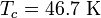$T_c =46.7 ~{\mathrm K}$ and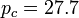$p_c = 27.7$ bar (Ref. 2 Table I), and the triple point at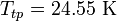$T_{tp} =24.55 ~{\mathrm K}$ and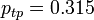$p_{tp} = 0.315$ bar (Ref. 2 Table II).

#### Crystallization line

The structural regularities along the crystallization line has been studied by way of path integral Monte Carlo simulations and the Ornstein-Zernike pair equation .